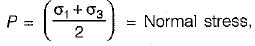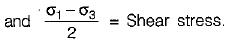Courses

# Test: Shear Strength of Soil - 2

## 10 Questions MCQ Test Soil Mechanics | Test: Shear Strength of Soil - 2

Description
This mock test of Test: Shear Strength of Soil - 2 for Civil Engineering (CE) helps you for every Civil Engineering (CE) entrance exam. This contains 10 Multiple Choice Questions for Civil Engineering (CE) Test: Shear Strength of Soil - 2 (mcq) to study with solutions a complete question bank. The solved questions answers in this Test: Shear Strength of Soil - 2 quiz give you a good mix of easy questions and tough questions. Civil Engineering (CE) students definitely take this Test: Shear Strength of Soil - 2 exercise for a better result in the exam. You can find other Test: Shear Strength of Soil - 2 extra questions, long questions & short questions for Civil Engineering (CE) on EduRev as well by searching above.
QUESTION: 1

### Unconfined compressive strength test is:

Solution:

The unconfined compression test is a special form of a tri-axial test in which the confining pressure is zero. The test can be conducted only on clayey soils which can stand without confinement.

QUESTION: 2

### The ratio, of the undisturbed shear strength to the remoulded shear strength in cohesive soil under undrained conditions is

Solution:

Ratio of undisturbed shear strength to the remoulded shear strength is called sensitivity.

QUESTION: 3

### The unconfined compressive strength of a pure clay soil is 100 kN/m2. What is the value of cohesion of the soil in kN/m2?

Solution:

Cohesion of soil = C = qu/2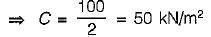QUESTION: 4

Match List-I (Field test) with List-ll (Useful for) and select the correct answer using the codes given below the lists:
List-I
A. Vane shear test
B. Standard penetration test
C. Static cone penetration test
D. Pressure meter test

List-ll
1. End bearing and skin friction resistance
2. In-situ stress-strain characteristics
3. Soft clay
4. Sandy deposits

Codes:
A B C D
(a) 4 2 1 3
(b) 3 4 1 2
(c) 4 3 2 1
(d) 3 4 2 1

Solution:

The shear modulus (G) can be determined in-situ by pressure meter. The original instrument was developed by Menard to overcome the problem of sampling disturbance. The instrument uses compressed gas (usually nitrogen). The results of test can be plotted as corrected pressure (p) against volume (v).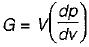The data can also be used for determination of undrained shear strength, angle of shearing resistance and other engineering properties of soil.

QUESTION: 5

A vane 20 cm long and 10 cm in diameter was pressed into a soft marine play at the bottom of a bore hole. Torque was applied gradually and failure occurred at 1000 kg-cm. The cohesion of the clay in kg/cm2 is

Solution:

Shear strength of clay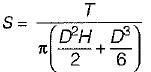⇒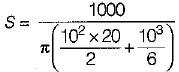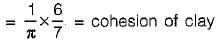QUESTION: 6

The results of a consolidated drained tri-axial shear test on a normally consolidated clay are shown in Figure below: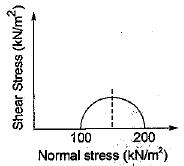The angle of internal friction is

Solution:

From the plot:
Major principal stress = σ1 = 200 kN/m2
Minor principal stress = σ3 = 100 kN/m2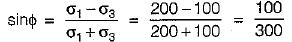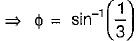QUESTION: 7

A and B are Skempton’s pore pressure parameters and Δσ1 and Δσ3 are incremental principal stresses. Skempton’s pore pressure equation is given by

Solution:
QUESTION: 8

A cylindrical specimen of saturated soil failed under an axial vertical stress of 100 kN/m2 when it was laterally unconfined. The failure plane was inclined to the horizontal plane at an angle of 45°. The values of cohesion and angle of internal friction for the soil are respectively.

Solution:

For an unconfined compression test:
S = C = 100/2 = 50kN/m2
= 0.05 N/mm2
A laterally unconfined compression test is conducted on soils which can stand without confinement. Also, for clay angle of internal friction = ϕ = 0°

QUESTION: 9

If the shearing stress is zero on two planes, then the angle between the two planes is

Solution:

The planes on which shearing stress is zero are major and minor principal planes. These two planes are inclined at 90° to each other.

QUESTION: 10

The state of stress on a plane inclined at an angle of 45° to the horizontal plane is represented on the Mohr’s circle by a point at which

Solution: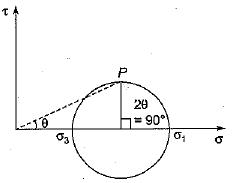A plane inclined at an angle θ = 45° to the horizontal plane is represented by the radius making an angle 2θ with the σ axis.
The state of stress is represented by Point ‘P'